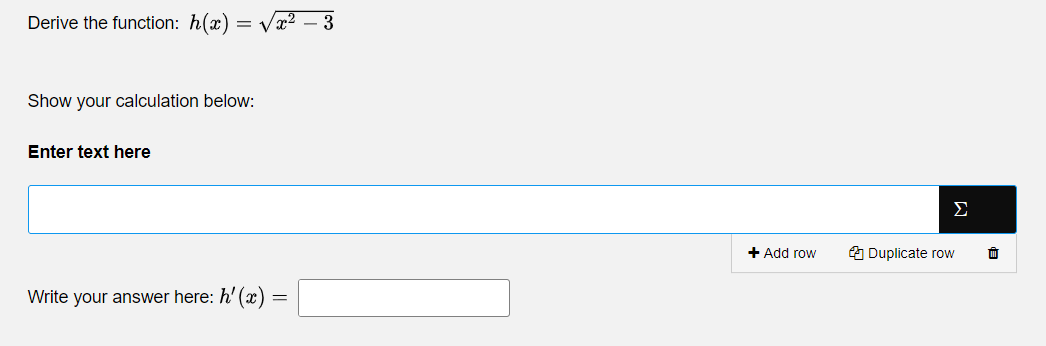# Question type - Math Entry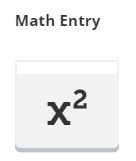Automatically marked question. The question is answered by entering a mathematical expression.

Math Entry is a question type where candidates can answer the question with mathematical symbols and formulas by using a palette. The question allows the Author to enter several correct answers related to an interaction element (the area where the candidate is to respond).

In the question's edit mode, you can do the following: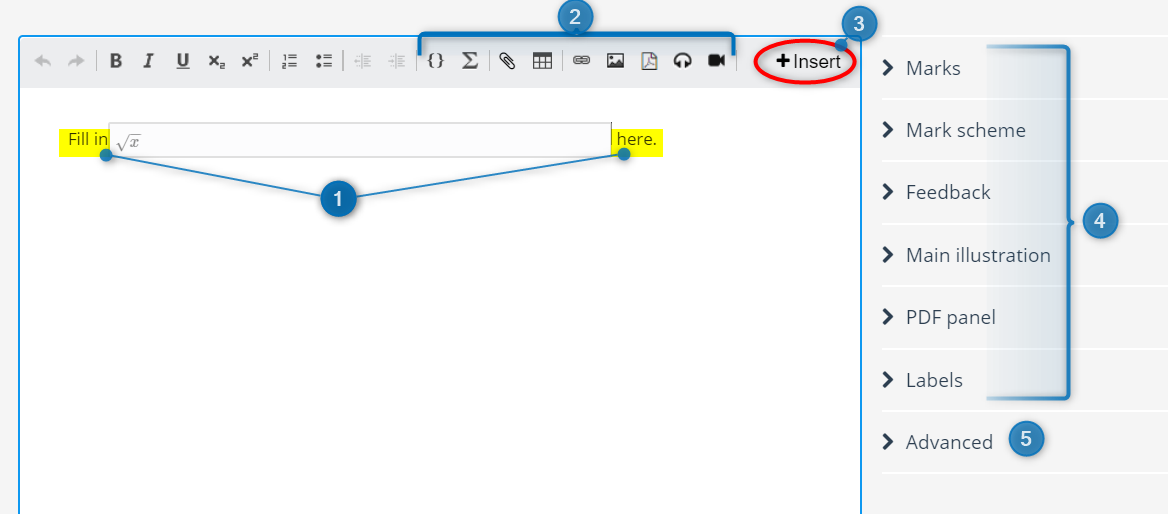1. Replace text with desired question text.

2. Add math (LaTex), files, tables, links, PDF, images and / or audio clips to the question text.

3. Insert multiple interaction elements by pressing +Insert.4. Set general options for the question type. Read more about general options for questions

• Allow unique responses only (Do not score duplicate answers): This applies to questions with more than one question element (interaction element). The purpose is to avoid awarding too many marks when a correct answer is repeated in the question elements.

Example: The question contains four math elements that each have the same fore correct answers defined.

This makes the question more flexible, allowing the candidates to choose which element they want to write the answer in. If the candidate fills in the same answer in all the elements, they can get marks for the correct answer for all the elements. With this setting enabled, the duplicate responses will not be counted as the correct answer more than once - the duplicates will be ignored.

## Specific options

Clicking on the interaction element gives you the specific options for the question type.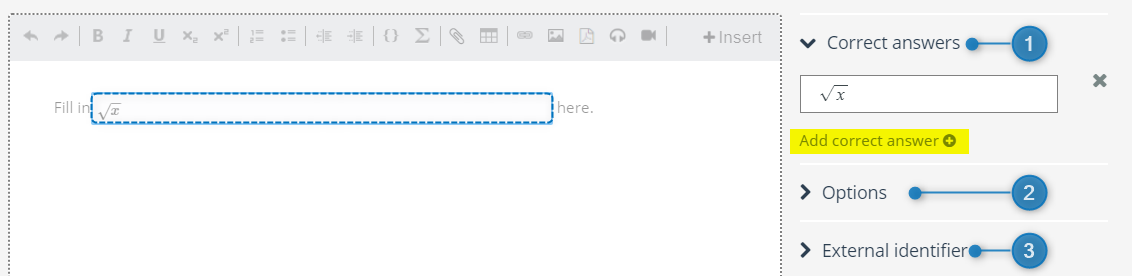Write the correct answer in the text box. The answer can contain both text and mathematical symbols and formulas.

Using a math palette consisting of two tabs, you can easily enter mathematical symbols and formulas:

 Basic Advanced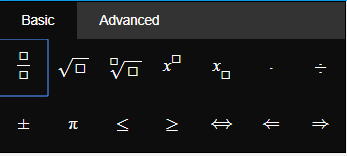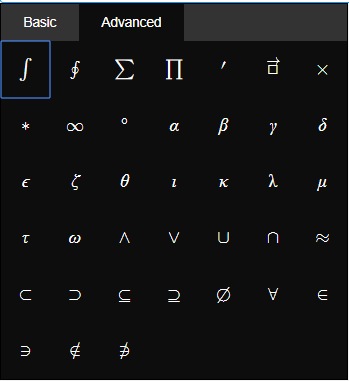It is also possible to add several correct answers to an interaction element by pressing Add correct answer.  Example: If you want the answer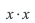to be accepted as answer in addition to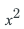.

### 2. Options

• Expected length (characters): Select the length of the selected interaction element. The length is chosen by entering the number of expected characters to be answered by the candidate.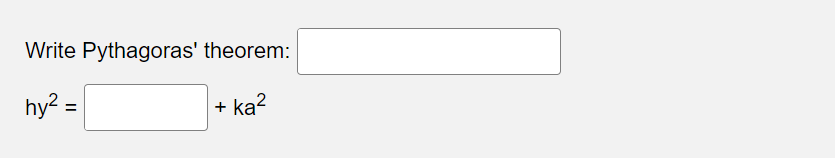Information: If you are writing an answer with one or more operands, you should create multiple versions of the correct answer as Inspera Assessment requires the answer to be identical. This means that if, for example, you have a space between the operands and the operator in the answer, the system require that the space is included in the candidates' answer.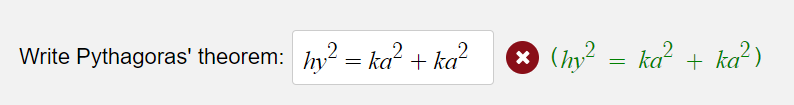Therefore, you may want to preview the question to make sure that the question will appear correctly to the candidates and that you have made sure that you have tested all possible ways to write correct answers.

### 3. External identifier

For information on external identifier, read general options for questions

Tip: If you want the candidates to be able to show the calculation that they have used to find the answer, you can combine Math Entry and the question type Math Working in a Composite question.